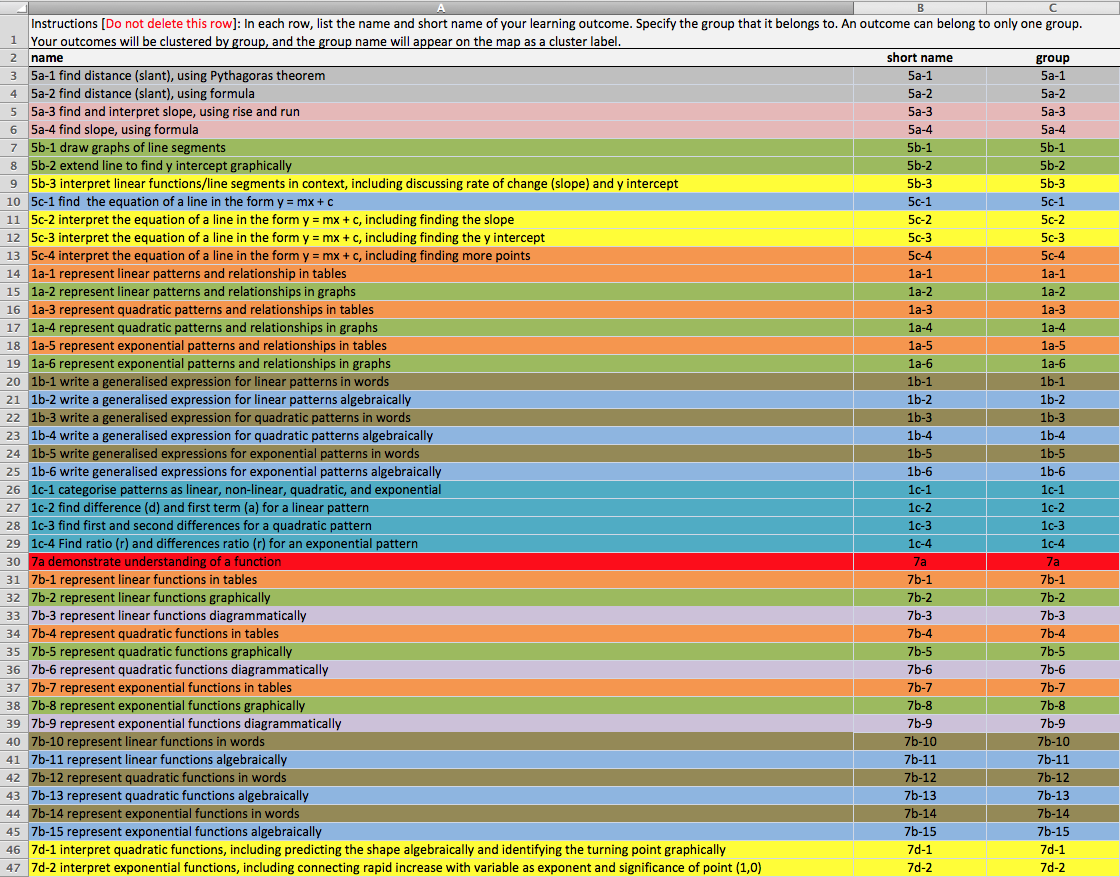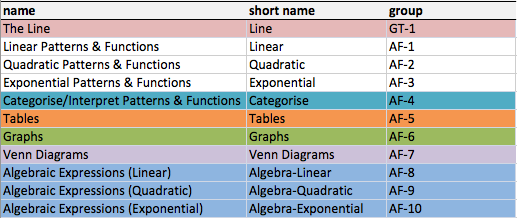# PD6-Post02

Design and Development of the Domain Model Artefacts

The 45 unpacked LOs from Phase 1 (Figure 11) were used to create the Rhumbl Maps artefact (Figure 14 and Figure 15). The 33 unified LOs from Phase 2 (Figure 16) were used to build the Mindomo artefact (Figure 17). Finally, the 22 linear LOs (Figure 18) helped construct the GAM AT artefact (Figure 19 and Figure 20).

Rhumbl Maps Domain Model
Rhumbl Maps was chosen as a domain model authoring tool because it is described as being capable of authoring learning outcomes for adaptive learning (Rhumbl, 2018). It achieves this by linking LOs to topics using three purpose built Excel spreadsheet templates for LOs, topics, and a matrix of LOs/topics. In simple terms, a topic, e.g. ‘Patterns and Relationships’, corresponds to a coarse-grained LO, e.g. investigate patterns and relationships (Figure 3) and a set of concepts are encapsulated in a topic (Sosnovsky & Brusilovsky, 2015). Linking LOs to topics is a non-conventional approach to adaptive learning. The traditional approach of concept-based adaptation links concepts to concepts (Sosnovsky & Brusilovsky, 2015). The first spreadsheet was populated with the 45 unpacked LOs from Phase 1 (Figure 11).Figure 11: Rhumbl Spreadsheet (45 Unpacked Learning Outcomes)

The second spreadsheet was populated with 11 topics formulated from the coarse-grained LOs (Figure 12). The first five topics relate to Figure 9 and Figure 10. The extra six topics (’Tables’, ‘Graphs’, etc.) allowed for a greater number of connections between topics and LOs, to allow for more effective adaptation (Sosnovsky & Brusilovsky, 2015)Figure 12: Rhumbl Spreadsheet (Topics)

The connections were created using a third spreadsheet, a matrix of topics and LOs (Figure 13). The digit ‘1’ in a cell indicates a connection. For example, LO 1a-2 is connected to three topics.Figure 13: Rhumbl Spreadsheet (Matrix)

This Excel spreadsheet matrix of topics and LOs is used by Rhumbl to generate two different views. The ‘Topic View’ is generated when the user clicks on a topic, e.g. Tables (Figure 14).Figure 14: Rhumbl Map Domain Model (Topic View)

The second display type, the ‘Outcome View’, is generated when the user clicks on a learning outcome, e.g. 7b-2 (Figure 15).Figure 15: Rhumbl Map Domain Model (Outcome View)

Mindomo Domain Model
To complete Phase 2, all pairs of LOs for patterns and functions from Phase 1 were unified. There were 12 such pairs, and with each pair counting as one LO, the result was 33 LOs (Figure 16). The reason for doing this is that one of the objectives of this research project was to connect Patterns (Strand AF.1) and Functions (Strand AF.7), which have strong ontological connections, so that these connections could be represented in a domain model. These 33 LOs were used to create the Mindomo domain model artefact, which emphasises the relationship between the concepts patterns and functions (Figure 17).Figure 16: The 33 Unified Learning Outcomes (Phase 2)Figure 17: Mindomo Domain Model

GAM AT Domain Model
The GAM AT software used to build the third and final domain model artefact was developed in 2017 (Craenen, 2017). The development of this tool built on the work that created the Adaptive Learning Authoring Tool (ALAT) (Boereboom, 2016; P. De Bra et al., 2016). ALAT in turn was an evolution of the GRAPPLE Authoring Tool (GAT) (P. De Bra et al., 2013; GRAPPLE, 2011). This domain model was created to represent the rich connections that exist between the concepts encapsulated in the strand topics Co-ordinate Geometry of the Line, Linear Patterns, and Linear Functions. GAM AT uses a hierarchy of fine-grained concepts. The completion of the Phase 3 process resulted in 22 LOs (Figure 18) that could then be represented as the 22 single word concepts required by the GAM AT functionality (Figure 19 and Figure 20).Figure 18: The 22 Linear Learning Outcomes (Phase 3)Figure 19: GAM AT Domain Model (List View)Figure 20: GAM AT Domain Model (Map View)